# Measurement other topics Temperature Scales Temperature Scales Temperature

• Slides: 17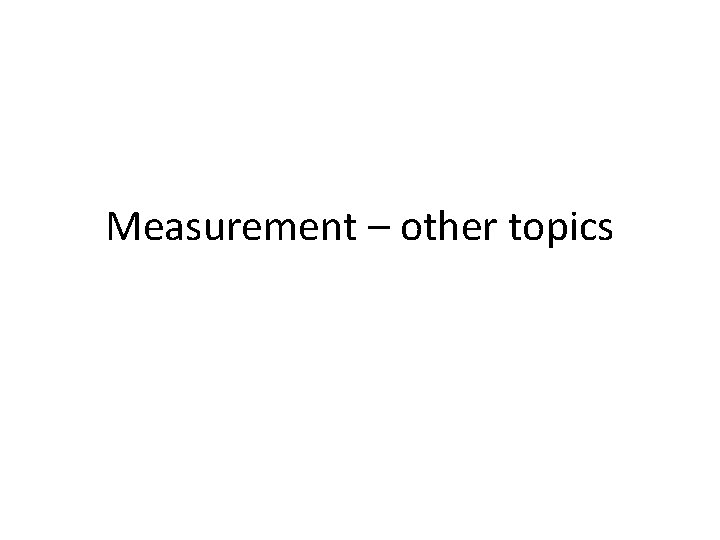Measurement – other topics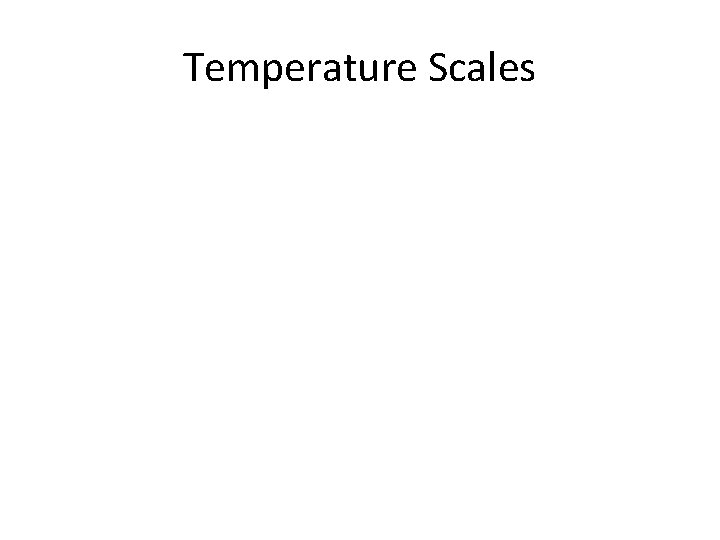Temperature ScalesTemperature ScalesTemperature Conversions • The Celsius scale is based on the freezing and boiling points of water at standard pressure.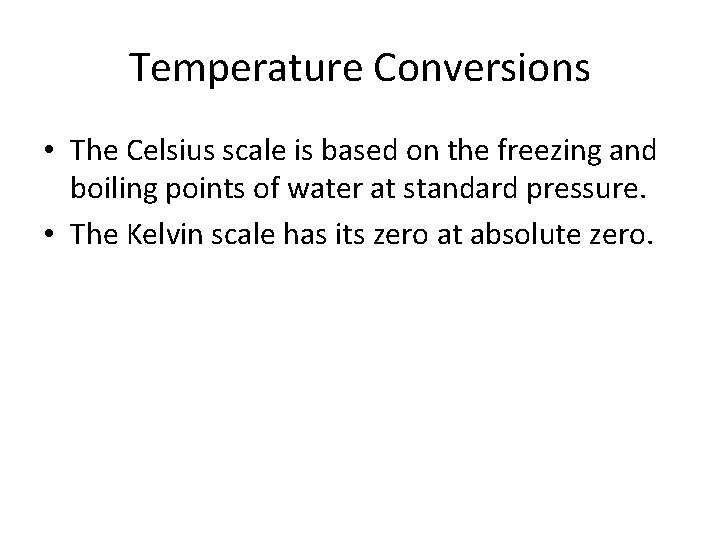Temperature Conversions • The Celsius scale is based on the freezing and boiling points of water at standard pressure. • The Kelvin scale has its zero at absolute zero.Temperature Conversions • The Celsius scale is based on the freezing and boiling points of water at standard pressure. • The Kelvin scale has its zero at absolute zero. • Kelvin units and Celsuis degrees are the same size.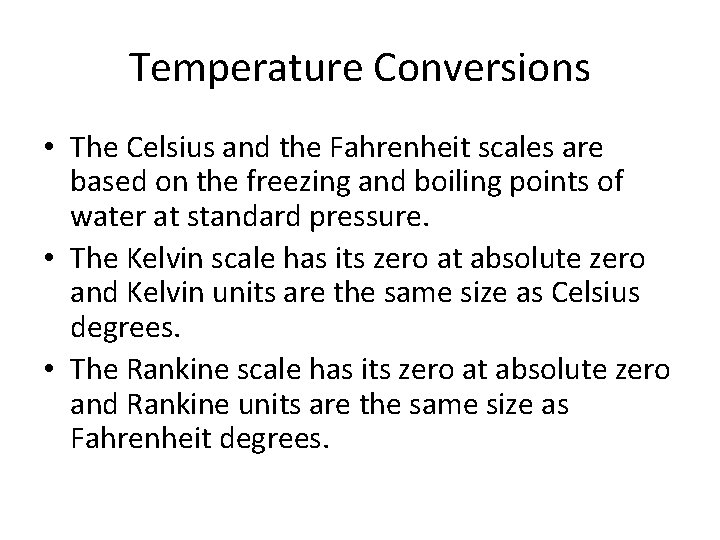Temperature Conversions • The Celsius and the Fahrenheit scales are based on the freezing and boiling points of water at standard pressure. • The Kelvin scale has its zero at absolute zero and Kelvin units are the same size as Celsius degrees. • The Rankine scale has its zero at absolute zero and Rankine units are the same size as Fahrenheit degrees.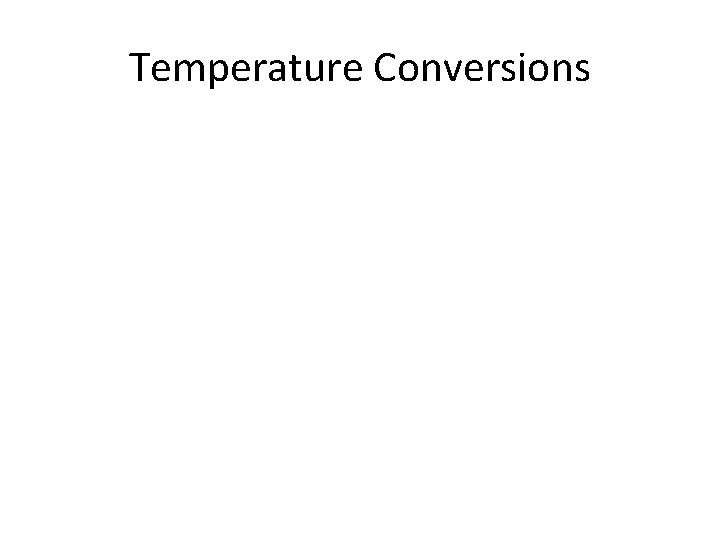Temperature ConversionsTemperature ConversionsTemperature Conversions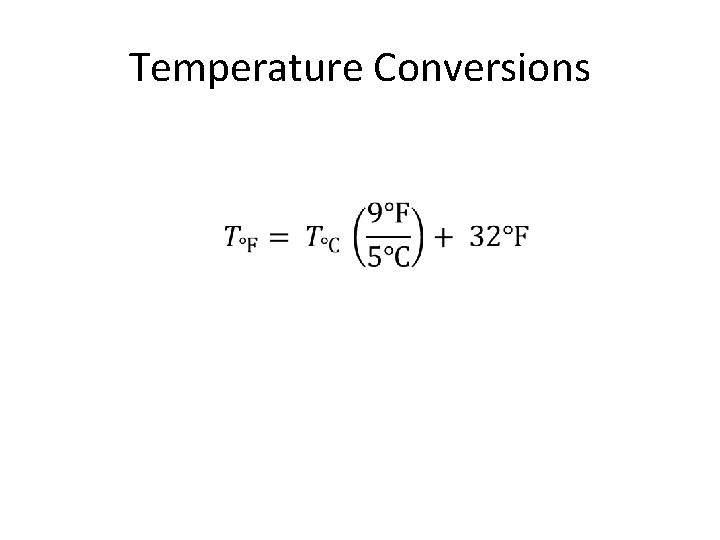Temperature Conversions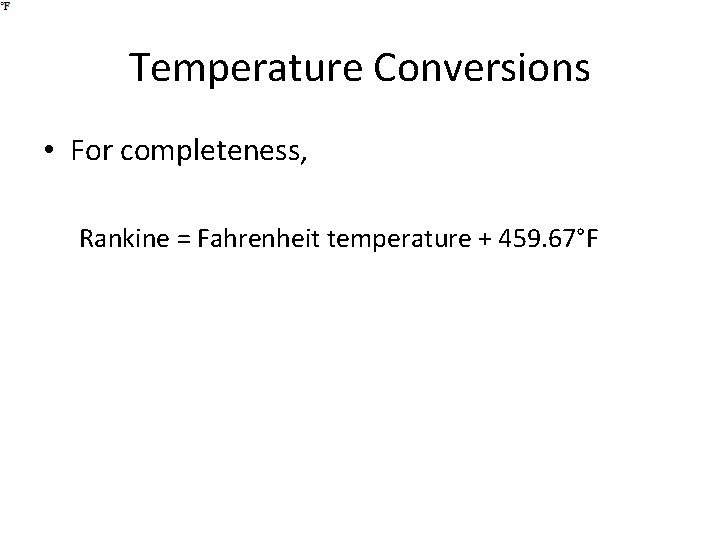Temperature Conversions • For completeness, Rankine = Fahrenheit temperature + 459. 67°F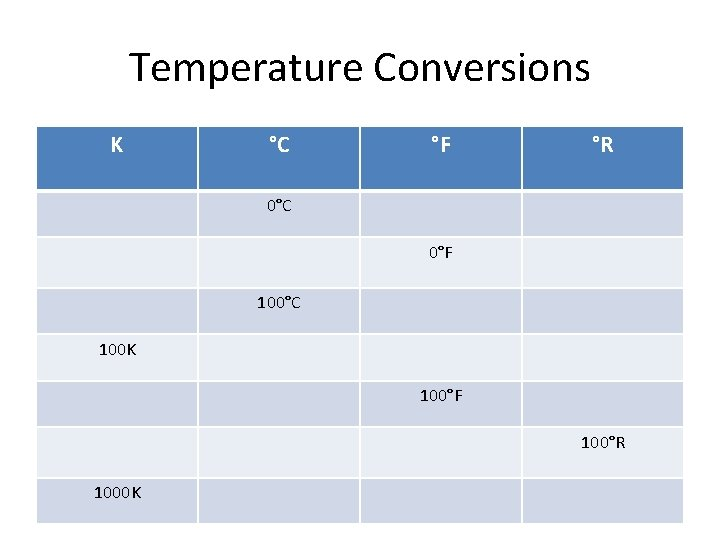Temperature Conversions K °C °F °R 0°C 0°F 100°C 100 K 100°F 100°R 1000 K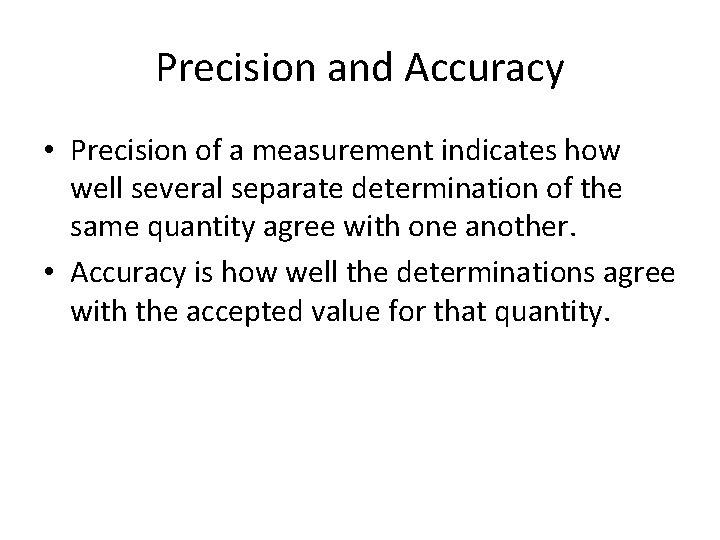Precision and Accuracy • Precision of a measurement indicates how well several separate determination of the same quantity agree with one another. • Accuracy is how well the determinations agree with the accepted value for that quantity.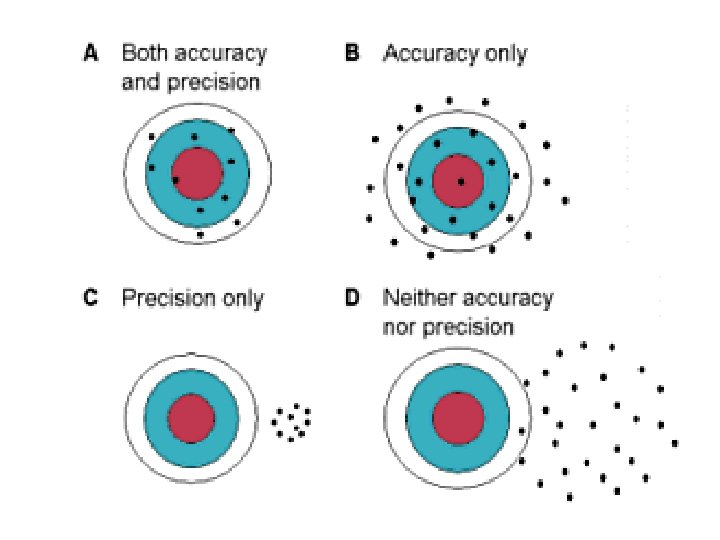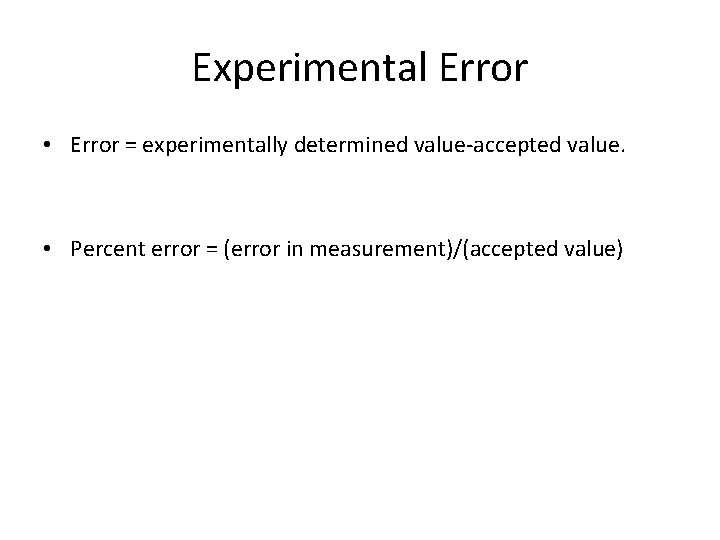Experimental Error • Error = experimentally determined value-accepted value. • Percent error = (error in measurement)/(accepted value)